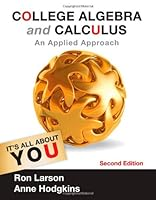# College Algebra and Calculus: An Applied Approach, 2nd Edition## Book Description

AND : AN APPLIED APPROACH, Second Edition provides a comprehensive resource for college algebra and applied courses. The mathematical concepts and applications are consistently presented in the same tone and pedagogy to promote confidence and a smooth transition from one course to the next. The consolidation of content for two courses in a single text saves instructors time in their course--and saves students the cost of an extra textbook.

Chapter 0. Fundamental Concepts of Algebra
Chapter 1. Equations and Inequalities
Chapter 2. Functions and
Chapter 3. Polynomial and Functions
Chapter 4. Exponential and Logarithmic Functions
Chapter 5. Systems of Equations and Inequalities
Chapter 6. Matrices and Determinants
Chapter 7. Limits and Derivatives
Chapter 8. Applications of the Derivative
Chapter 9. Further Applications of the Derivative
Chapter 10. Derivatives of Exponential and Logarithmic Functions
Chapter 11. and Its Applications
Chapter 12. Techniques of Integration
Chapter 13. Functions of Several Variables
Chapter 14. Trigonometric Functions (WEB)
Chapter 15. Series and Taylor Polynomials (WEB)
Chapter 16. Probability (WEB)

## Book Details

• Title: College Algebra and Calculus: An Applied Approach, 2nd Edition
• Author: ,
• Length: 1136 pages
• Edition: 2
• Language: English
• Publisher:
• Publication Date: 2012-01-01
• ISBN-10: 1133105068
• ISBN-13: 9781133105060